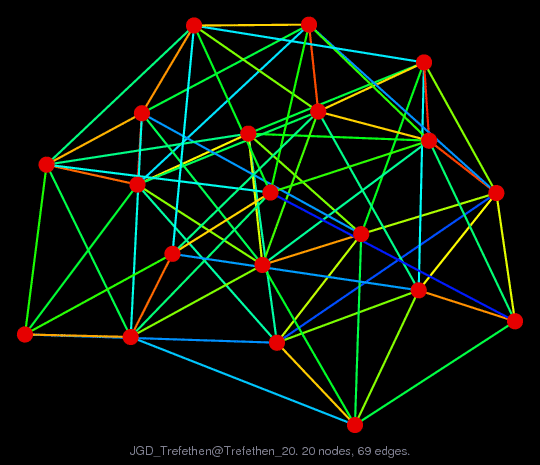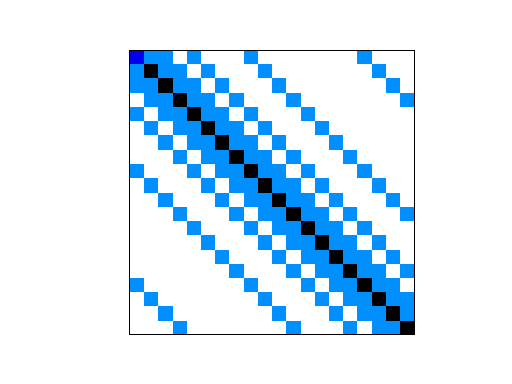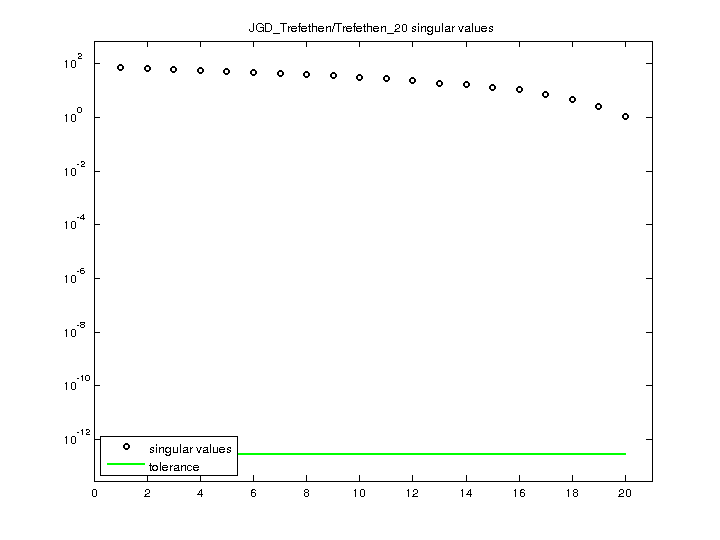Matrix: JGD_Trefethen/Trefethen_20

Description: Diagonal matrices with primes, Nick Trefethen, Oxford Univ.(undirected graph drawing)• Matrix group: JGD_Trefethen
• download as a MATLAB mat-file, file size: 1 KB. Use UFget(2204) or UFget('JGD_Trefethen/Trefethen_20') in MATLAB.

 Matrix properties number of rows 20 number of columns 20 nonzeros 158 structural full rank? yes structural rank 20 # of blocks from dmperm 1 # strongly connected comp. 1 explicit zero entries 0 nonzero pattern symmetry symmetric numeric value symmetry symmetric type integer structure symmetric Cholesky candidate? yes positive definite? yes

 author N. Trefethen editor J.-G. Dumas date 2008 kind combinatorial problem 2D/3D problem? no

Notes:

```Diagonal matrices with primes, Nick Trefethen, Oxford Univ.
From Jean-Guillaume Dumas' Sparse Integer Matrix Collection,
http://ljk.imag.fr/membres/Jean-Guillaume.Dumas/simc.html

Problem 7 of the Hundred-dollar, Hundred-digit Challenge Problems,
SIAM News, vol 35, no. 1.

7. Let A be the 20,000 x 20,000 matrix whose entries are zero
everywhere except for the primes 2, 3, 5, 7, . . . , 224737 along the
main diagonal and the number 1 in all the positions A(i,j) with
|i-j| = 1,2,4,8, . . . ,16384.  What is the (1,1) entry of inv(A)?

http://www.siam.org/news/news.php?id=388

Filename in JGD collection: Trefethen/trefethen_20.sms
```

 Ordering statistics: result nnz(chol(P*(A+A'+s*I)*P')) with AMD 147 Cholesky flop count 1.3e+03 nnz(L+U), no partial pivoting, with AMD 274 nnz(V) for QR, upper bound nnz(L) for LU, with COLAMD 174 nnz(R) for QR, upper bound nnz(U) for LU, with COLAMD 207

 SVD-based statistics: norm(A) 71.5124 min(svd(A)) 1.13352 cond(A) 63.0886 rank(A) 20 sprank(A)-rank(A) 0 null space dimension 0 full numerical rank? yes

 singular values (MAT file): click here SVD method used: s = svd (full (A)) status: ok